• Slides: 28Comunicaciones Digitales Avanzadas EL 7041 Comunicaciones Digitales Avanzadas César Azurdia Meza, Ph. D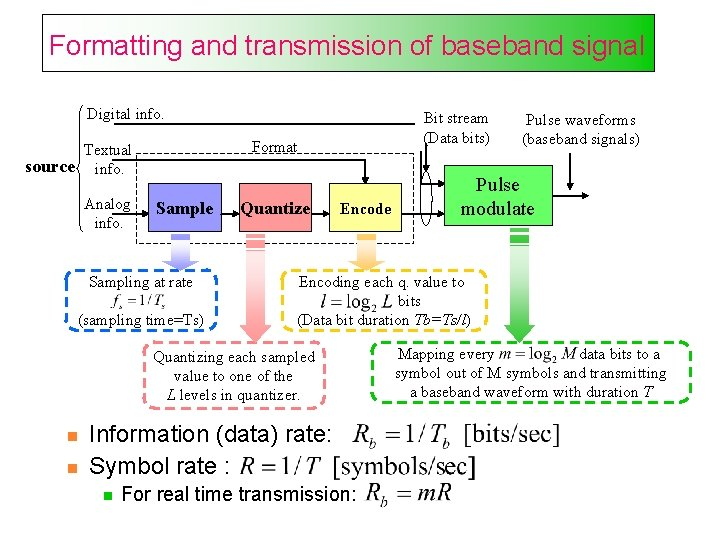Formatting and transmission of baseband signal Digital info. Format Textual source info. Analog info. Bit stream (Data bits) Sample Sampling at rate (sampling time=Ts) Quantize Encode Information (data) rate: Symbol rate : Pulse modulate Encoding each q. value to bits (Data bit duration Tb=Ts/l) Quantizing each sampled value to one of the L levels in quantizer. Pulse waveforms (baseband signals) For real time transmission: Mapping every data bits to a symbol out of M symbols and transmitting a baseband waveform with duration TQuantization example amplitude x(t) 111 3. 1867 110 2. 2762 101 1. 3657 100 0. 4552 Quant. levels boundaries 011 -0. 4552 010 -1. 3657 001 -2. 2762 xq(n. Ts): quantized values x(n. Ts): sampled values 000 -3. 1867 Ts: sampling time PCM codeword t 110 111 110 100 011 PCM sequenceExample of M-ary PAM Assuming real time transmission and equal energy per transmission data bit for binary-PAM and 4 -ary PAM: • 4 -ary: T=2 Tb and Binary: T=Tb • Binary PAM (rectangular pulse) 4 -ary PAM (rectangular pulse) 3 B A. ‘ 1’ T T T ‘ 10’ ‘ 0’ -A. -3 B T -B ‘ 00’ B ‘ 01’ T ‘ 11’ TExample of M-ary PAM … 0 Ts 2. 2762 V 0 Tb 2 Tb 3 Tb 4 Tb 5 Tb 6 Tb 1 0 0 1. 3657 V T 1 0 2 T T 1 3 T 0 4 T 2 T 5 T 1 6 T 3 T Rb=1/Tb=3/Ts R=1/Tb=3/Ts Rb=1/Tb=3/Ts R=1/T=1/2 Tb=3/2 Ts=1. 5/Ts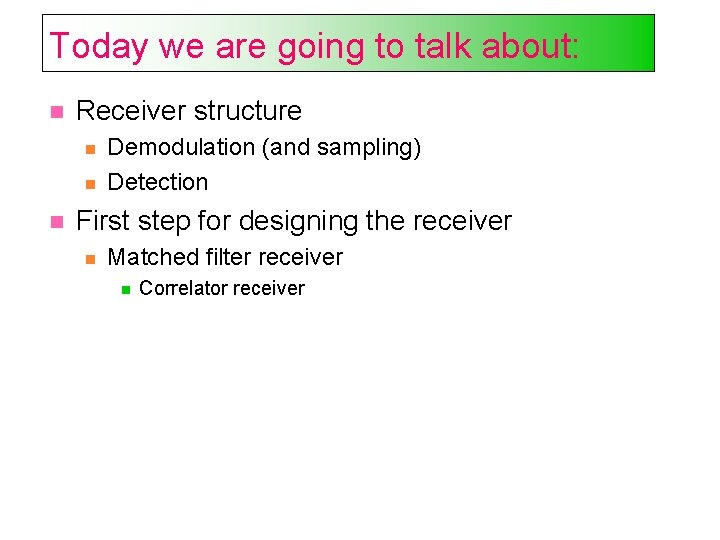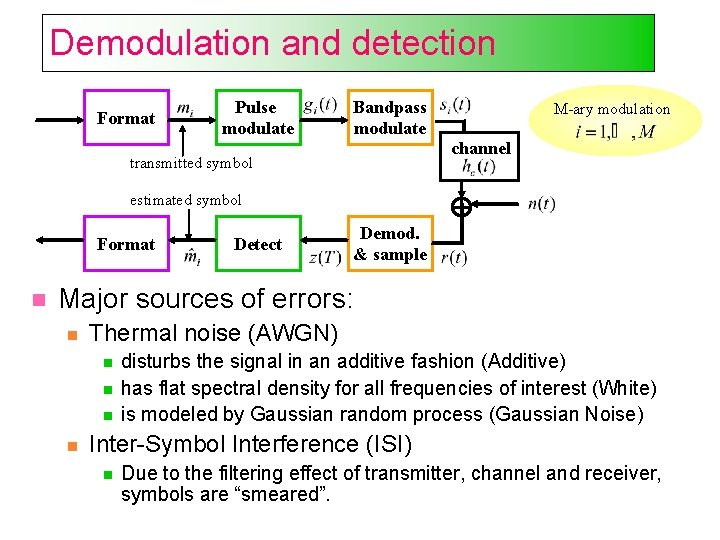Demodulation and detection Format Pulse modulate Bandpass modulate transmitted symbol M-ary modulation channel estimated symbol Format Detect Demod. & sample Major sources of errors: Thermal noise (AWGN) disturbs the signal in an additive fashion (Additive) has flat spectral density for all frequencies of interest (White) is modeled by Gaussian random process (Gaussian Noise) Inter-Symbol Interference (ISI) Due to the filtering effect of transmitter, channel and receiver, symbols are “smeared”.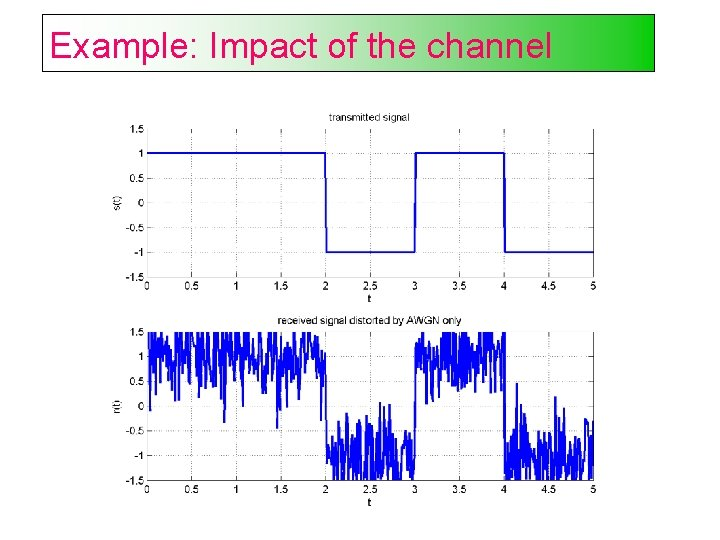Example: Impact of the channel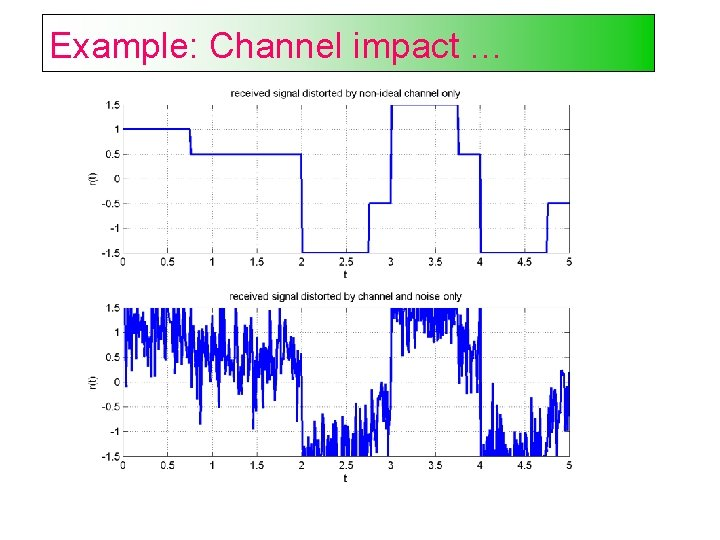Example: Channel impact …Receiver tasks Demodulation and sampling: Waveform recovery and preparing the received signal for detection: Improving the signal power to the noise power (SNR) using matched filter Reducing ISI using equalizer Sampling the recovered waveform Detection: Estimate the transmitted symbol based on the received sampleReceiver structure Step 1 – waveform to sample transformation Step 2 – decision making Demodulate & Sample Frequency down-conversion For bandpass signals Received waveform Receiving filter Detect Equalizing filter Threshold comparison Compensation for channel induced ISI Baseband pulse (possibly distored) Baseband pulse Sample (test statistic)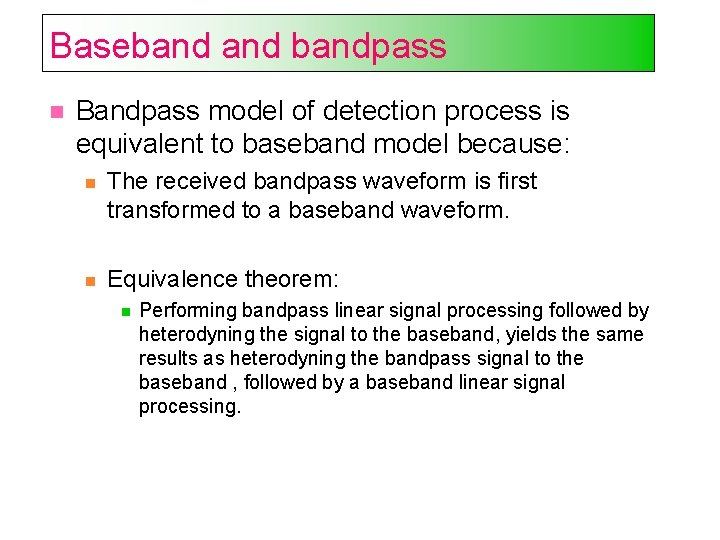Basebandpass Bandpass model of detection process is equivalent to baseband model because: The received bandpass waveform is first transformed to a baseband waveform. Equivalence theorem: Performing bandpass linear signal processing followed by heterodyning the signal to the baseband, yields the same results as heterodyning the bandpass signal to the baseband , followed by a baseband linear signal processing.Steps in designing the receiver Find optimum solution for receiver design with the following goals: 1. Maximize SNR 2. Minimize ISI Steps in design: Model the received signal Find separate solutions for each of the goals. First, we focus on designing a receiver which maximizes the SNR.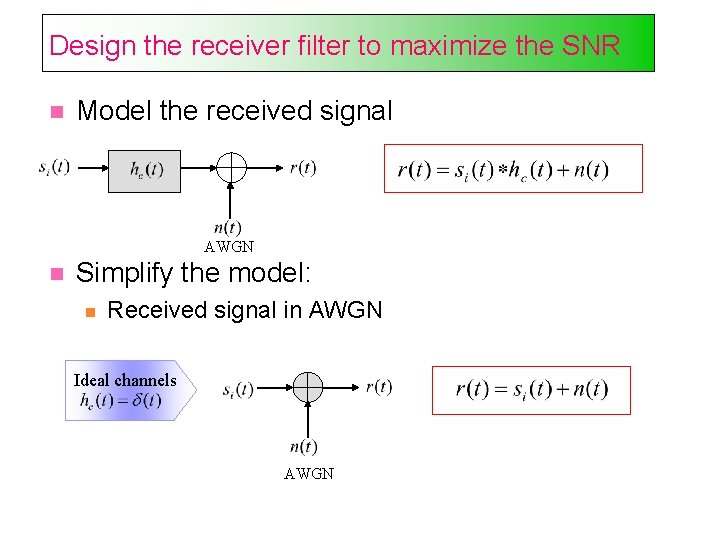Design the receiver filter to maximize the SNR Model the received signal AWGN Simplify the model: Received signal in AWGN Ideal channels AWGN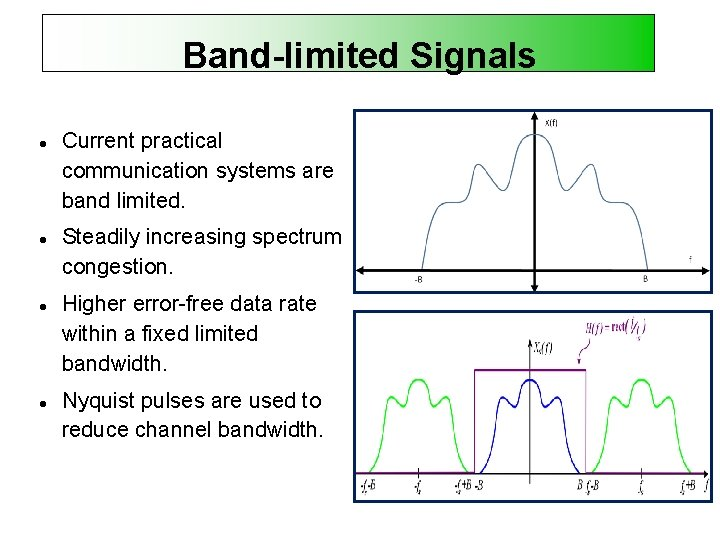Band-limited Signals Current practical communication systems are band limited. Steadily increasing spectrum congestion. Higher error-free data rate within a fixed limited bandwidth. Nyquist pulses are used to reduce channel bandwidth.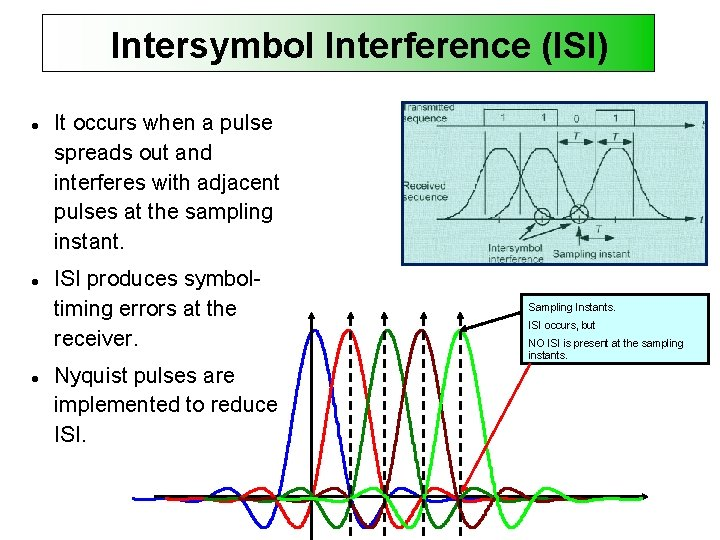Intersymbol Interference (ISI) It occurs when a pulse spreads out and interferes with adjacent pulses at the sampling instant. ISI produces symboltiming errors at the receiver. Nyquist pulses are implemented to reduce ISI. Sampling Instants. ISI occurs, but NO ISI is present at the sampling instants.Channel Filter • Filtro implementado para limitar el ancho de bando en base a las especificaciones del canal. q Pass-band q Base-band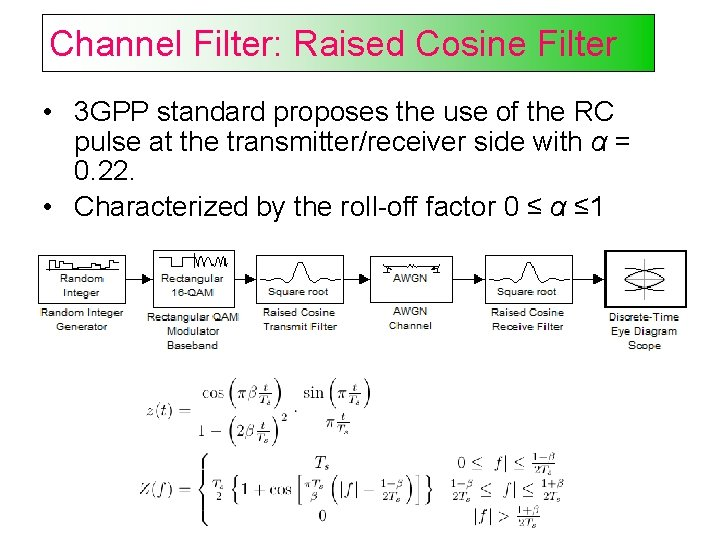Channel Filter: Raised Cosine Filter • 3 GPP standard proposes the use of the RC pulse at the transmitter/receiver side with α = 0. 22. • Characterized by the roll-off factor 0 ≤ α ≤ 1Channel Filter: Raised Cosine Filter Respuesta al Impulso Respuesta en Frecuencia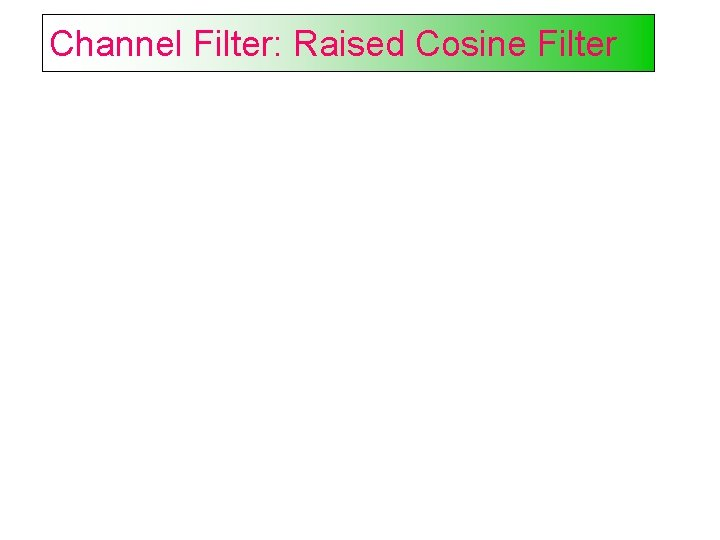Channel Filter: Raised Cosine FilterMatched Filter: Root Raised Cosine Filter • According to the 3 GPP standard, the impulse response of the RRC pulse is given as: Time Domain Root Raised Cosine PulseMatched filter receiver Problem: Design the receiver filter such that the SNR is maximized at the sampling time when is transmitted. Solution: The optimum filter, is the Matched filter, given by which is the time-reversed and delayed version of the conjugate of the transmitted signal 0 T t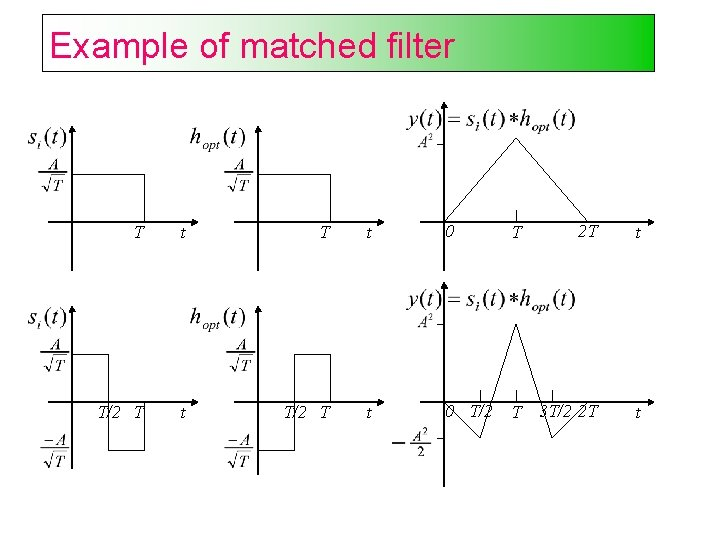Example of matched filter T t 0 T 2 T t T/2 T t 0 T/2 T 3 T/2 2 T tProperties of the matched filter The Fourier transform of a matched filter output with the matched signal as input is, except for a time delay factor, proportional to the ESD of the input signal. The output signal of a matched filter is proportional to a shifted version of the autocorrelation function of the input signal to which the filter is matched. The output SNR of a matched filter depends only on the ratio of the signal energy to the PSD of the white noise at the filter input. Two matching conditions in the matched-filtering operation: spectral phase matching that gives the desired output peak at time T. spectral amplitude matching that gives optimum SNR to the peak value.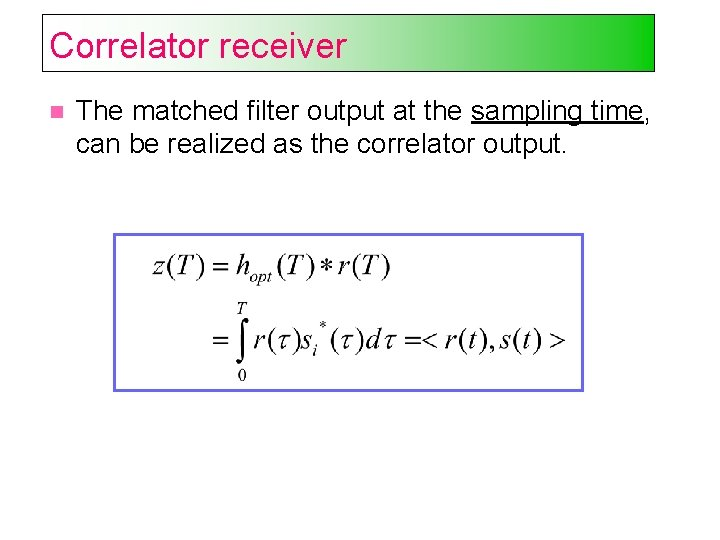Correlator receiver The matched filter output at the sampling time, can be realized as the correlator output.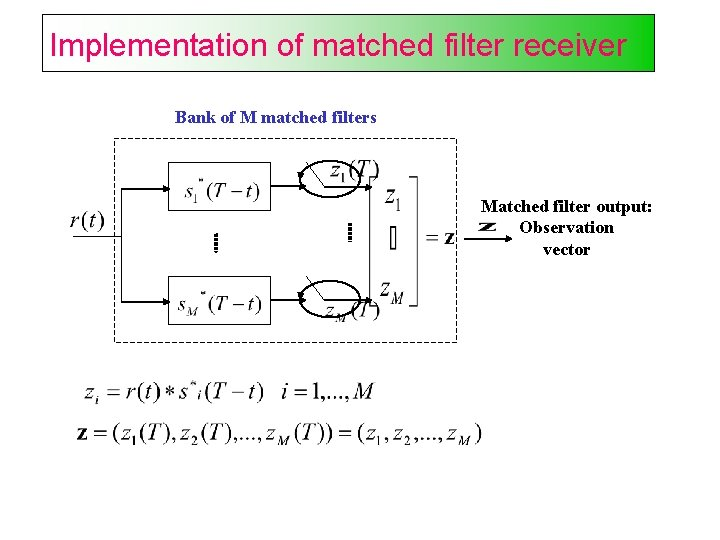Implementation of matched filter receiver Bank of M matched filters Matched filter output: Observation vector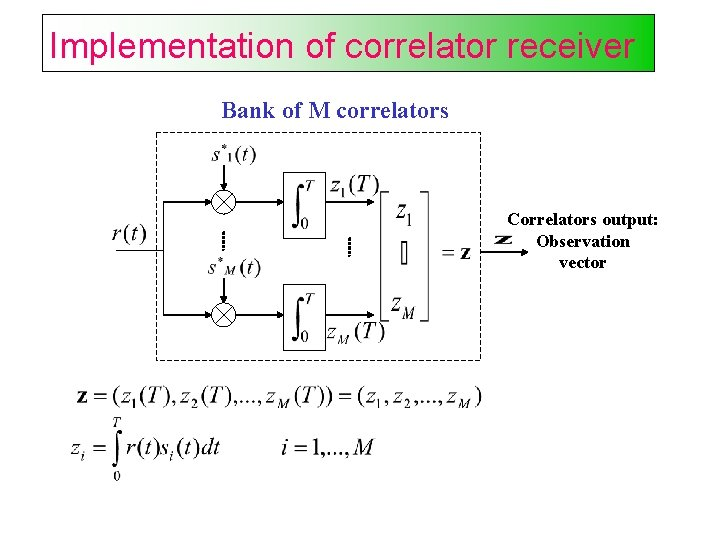Implementation of correlator receiver Bank of M correlators Correlators output: Observation vectorImplementation example of matched filter receivers Bank of 2 matched filters 0 T t 0 T 0 0 T t T